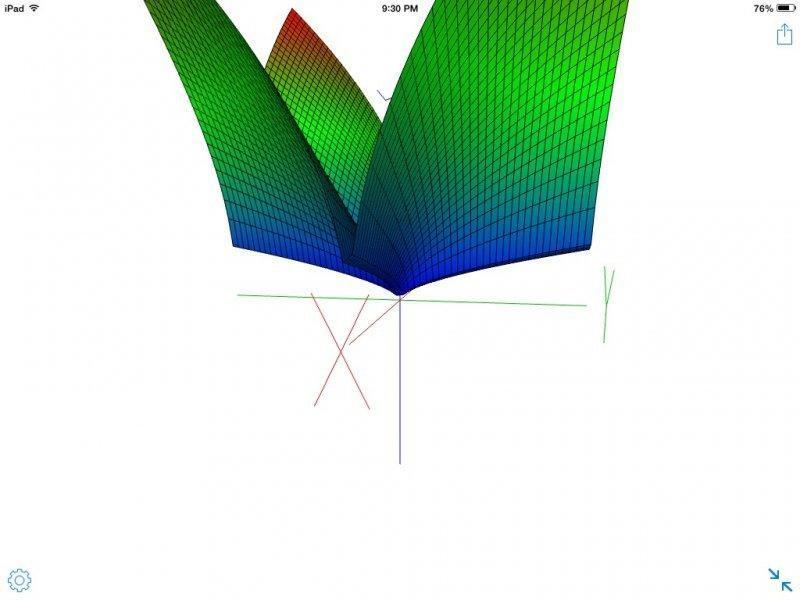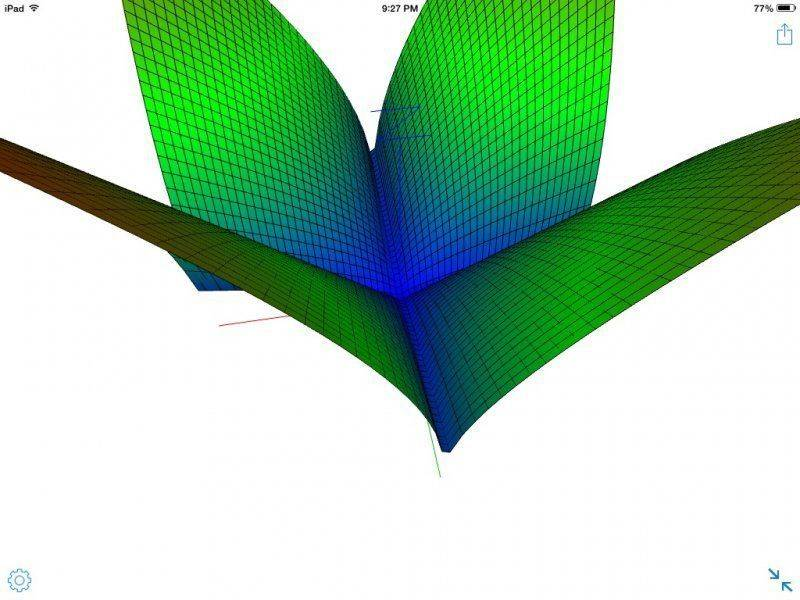# I need an example of a function.

• paalfis

#### paalfis

Member warned about not using the homework template
Give an example of a function f:R2-->R , continuous in (0,0), with partial derivatives in (0,0), but not differentiable in (0,0).

I was thinking in something like f(x,y)=IxyI

What is the definition of continuous function at a point like (0, 0) ?

limit as (x,y)->(0,0) of If(x,y)-f(0,0)I=0

I know what this properties mean, I just can't think of a function with partial derivatives in (0,0) but not differentiable in that point.

Actually, I think that the function f (x , y) = x1/3y2/3+y1/3x2/3 works fine, am I right?

Yeah that seems to work. I plotted it here.